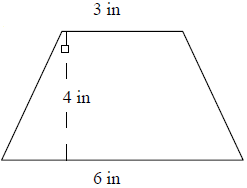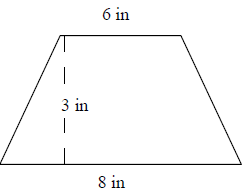# Finding the area of a trapezoid on a grid by using triangles and rectangles

In this lesson we find areas of trapezoids on grids by using triangles and rectangles. The trapezoids are decomposed into triangles and rectangles and their areas found. The sum of these areas gives the area of the trapezoid on the grid.

We know the area of a triangle = $\frac{1}{2}$ b × h and

area of a rectangle = l × w

Find the area of the following trapezoid by using triangles and rectangles.### Solution

Step 1:

Area of Right Triangle = $\frac{1}{2}$ × b × h; b = base; h = height.

Area of 2 right triangles = 2 × $\frac{1}{2}$ × b × h = 1.5 × 4 = 6 square in.

Step 2:

Area of Rectangle = l × w = 4 × 3 = 12 square in

Step 3:

Area of trapezoid = Area of triangles + Area of rectangle

= 6 + 12

= 18 square in

Find the area of the following trapezoid by using triangles and rectangles.### Solution

Step 1:

Area of Right Triangle = $\frac{1}{2}$ × b × h; b = base; h = height.

Area of 2 right triangles = 2 × $\frac{1}{2}$ × b × h = 1 × 3 = 3 square in.

Step 2:

Area of Rectangle = l × w = 6 × 3 = 18 square in

Step 3:

Area of trapezoid = Area of triangles + Area of rectangle

= 3 + 18

= 21 square in